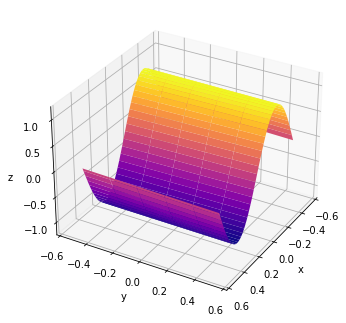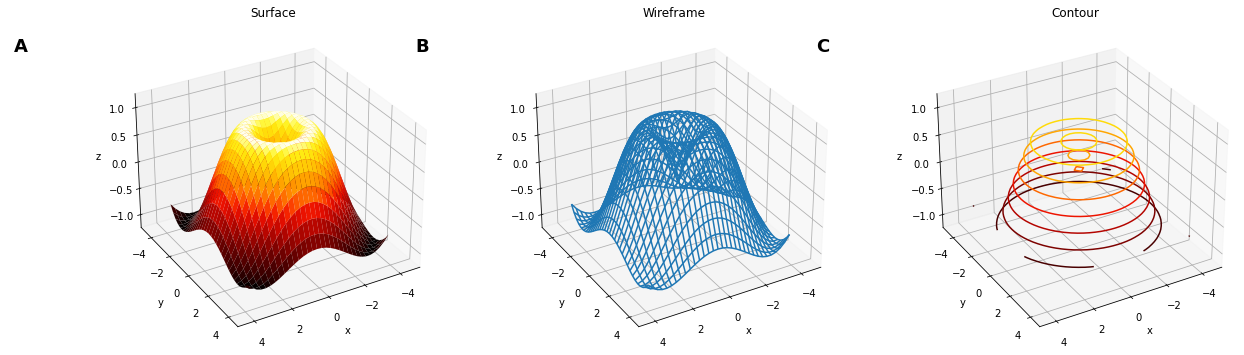# Surface#

Title
Surface Element
Dependencies
Matplotlib
Backends
Matplotlib
Plotly
```import numpy as np
import holoviews as hv
from holoviews import opts

hv.extension('matplotlib')
````Surface` is used for a set of gridded points whose associated value dimension represents samples from a continuous surface. `Surface` is equivalent to an `Image` type and supports all the same data formats, including simply NumPy arrays with associated `bounds` and other gridded data formats such as xarray.

Rendering a large can often be quite expensive, using `rstride` and `cstride` we can draw a coarser surface. We can also control the `azimuth`, `elevation` and `distance` as plot options to control the camera angle:

```surface = hv.Surface(np.sin(np.linspace(0,100*np.pi*2,10000)).reshape(100,100))

surface.opts(opts.Surface(azimuth=30, elevation=30, rstride=20, cstride=2, cmap='plasma'))
```In addition to a simple surface plots, the matplotlib surface plot also supports other related `plot_type` modes including `'wireframe'` and `'contour'` plots:

```xs = np.arange(-4, 4, 0.25)
ys = np.arange(-4, 4, 0.25)
X, Y = np.meshgrid(xs, ys)
R = np.sqrt(X**2 + Y**2)
Z = np.sin(R)

surface = hv.Surface((xs, ys, Z), label='Surface')
wireframe = surface.relabel('Wireframe').opts(plot_type='wireframe')
contour = surface.relabel('Contour').opts(plot_type='contour')

(surface + wireframe + contour).opts(
opts.Layout(fig_size=150, hspace=0.1),
opts.Surface(azimuth=60, cmap='fire'))
```For full documentation and the available style and plot options, use `hv.help(hv.Surface).`

This web page was generated from a Jupyter notebook and not all interactivity will work on this website. Right click to download and run locally for full Python-backed interactivity.